## Example Questions

← Previous 1 3

### Example Question #1 : Right Triangles

A triangle has three internal angles of 75, 60, and x. What is x?

110

60

75

45

90

45

Explanation:

The internal angles of a triangle must add up to 180. 180 - 75 -60= 45.

### Example Question #2 : Right Triangles

Quantitative Comparison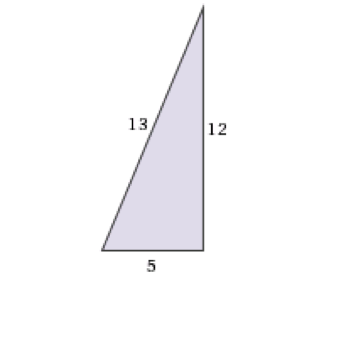Column A

Area

Column B

Perimeter




Column B is greater

Column A is greater

Cannot be determined

Column A and B are equal

Column A and B are equal

Explanation:

To find the perimeter, add up the sides, here 5 + 12 + 13 = 30. To find the area, multiply the two legs together and divide by 2, here (5 * 12)/2 = 30.

### Example Question #1 : Right Triangles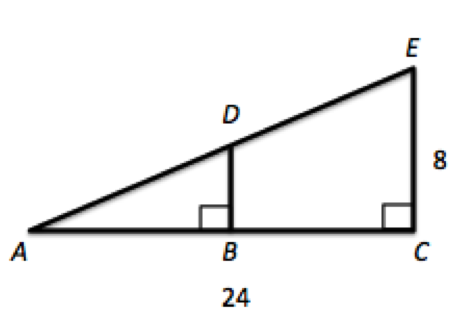Given triangle ACE where B is the midpoint of AC, what is the area of triangle ABD?

48

96

72

24

24

Explanation:

If B is a midpoint of AC, then we know AB is 12. Moreover, triangles ACE and ABD share angle DAB and have right angles which makes them similar triangles. Thus, their sides will all be proportional, and BD is 4. 1/2bh gives us 1/* 12 * 4, or 24.

### Example Question #91 : Geometry

What is the area of a right triangle with hypotenuse of 13 and base of 12?

30

25

156

60

78

30

Explanation:

Area = 1/2(base)(height). You could use Pythagorean theorem to find the height or, if you know the special right triangles, recognize the 5-12-13. The area = 1/2(12)(5) = 30.

### Example Question #1 : Right Triangles

Quantitative Comparison

Quantity A: the area of a right triangle with sides 10, 24, 26

Quantity B: twice the area of a right triangle with sides 5, 12, 13

Quantity A is greater.

The relationship cannot be determined from the information given.

Quantity B is greater.

The two quantities are equal.

Quantity A is greater.

Explanation:

Quantity A: area = base * height / 2 = 10 * 24 / 2 = 120

Quantity B: 2 * area = 2 * base * height / 2 = base * height = 5 * 12 = 60

Therefore Quantity A is greater.

### Example Question #1 : Right Triangles

Quantitative Comparison

Quantity A: The area of a triangle with a height of 6 and a base of 7

Quantity B: Half the area of a trapezoid with a height of 6, a base of 6, and another base of 10

Quantity A is greater.

The relationship cannot be determined from the information given.

The two quantities are equal.

Quantity B is greater.

Quantity B is greater.

Explanation:

Quantity A: Area = 1/2 * b * h = 1/2 * 6 * 7 = 42/2 = 21

Quantity B: Area = 1/2 * (b1 + b2) * h = 1/2 * (6 + 10) * 6 = 48

Half of the area = 48/2 = 24

Quantity B is greater.

### Example Question #42 : Triangles

The radius of the circle is 2. What is the area of the shaded equilateral triangle?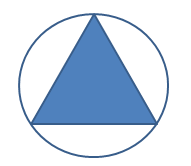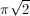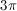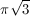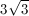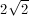Explanation:

This is easier to see when the triangle is divided into six parts (blue). Each one contains an angle which is half of 120 degrees and contains a 90 degree angle. This means each triangle is a 30/60/90 triangle with its long side equal to the radius of the circle. Knowing that means that the height of each triangle is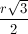and the base is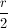.

Applying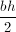and multiplying by 6 gives).### Example Question #8 : Right Triangles

Quantitative Comparison

Quantity A: The area of a triangle with a perimeter of 34

Quantity B: 30

Quantity B is greater.

The two quantities are equal.

The relationship cannot be determined from the information given.

Quantity A is greater.

The relationship cannot be determined from the information given.

Explanation:

A triangle with a fixed perimeter does not have to have a fixed area. For example, a triangle with sides 3, 4, and 5 has a perimeter of 12 and an area of 6. A triangle with sides 4, 4, and 4 also has a perimeter of 12 but not an area of 6. Thus the answer cannot be determined.

### Example Question #9 : Right Triangles

2 triangles are similar

Triangle 1 has sides  6, 8, 10

Triangle 2 has sides 5 , 3, x

find x

2

9

4

5

12

4

Explanation:

Draw the triangles

Triangle 1 is a 6,8,10 right triangle with 10 as the hypotenuse

Triangle 2: 3 is half of 6, 5 is half of 10; x must be half of 8

### Example Question #10 : Right Triangles

Given the diagram, indicate if Quantity A is larger, Quantity B is larger, if they are equal, or if there is not enough information given to determine the relationship.

Quantity A:Quantity B: 7.5

The two quantities are equal.

The relaionship cannot be determined from the information given.

Quantity B is greater.

Quantity A is greater.

Quantity A is greater.

Explanation:

Since this is a 30-60-90 triangle, we know that the length of the side opposite the 60 degree angle is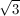times the side opposite the 30 degree angle. Thus,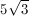, which is about 8.66. This is larger than 7.5.

← Previous 1 3

Tired of practice problems?

Try live online GRE prep today.